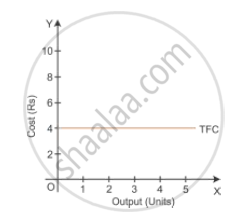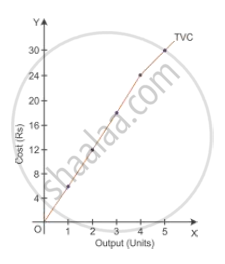# Define cost. State the behaviour of (a) Total Fixed Cost and (b) Total Variable Cost as output is increased. - Economics

Define cost. State the behaviour of (a) Total Fixed Cost and (b) Total Variable Cost as output is increased.

#### Solution

Cost is the total expenditure incurred in producing a commodity. In economics, it is the total of actual expenditure incurred on inputs (i.e. explicit cost) and the imputed valued of inputs supplied by owners (i.e. implicit cost).

(a) Total Fixed Cost (TFC):- Total of expenditure incurred by the producer on the purchase or hiring of fixed factors of production. It does not change with change in quantity of output and remains the same whether the output is zero or maximum. For example, the cost of plant and machinery and the wages of permanent staff.

 Output (units) TFC (in Rupees) 0 4 1 4 2 4 3 4 4 4 5 4Analysing the TFC schedule and the figure, we can say that TFC remains constant irrespective of the output levels. Therefore, in the short run, TFC is a horizontal curve parallel to the x-axis.

(b) Total Variable Cost (TVC):- Variable cost is the expenditure incurred by the producer on the use of variable factors of production. Variable cost changes with change in quantity of output. For example, the cost of raw materials and fuel expenses.

 Output (units) TVC (in Rupees) 0 0 1 6 2 12 3 18 4 24 5 30Concept: Basic Concepts of Cost
Is there an error in this question or solution?
2015-2016 (March) Foreign Set 1

Share